# Possibilities of Canvas.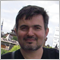6758

Demonstration of the possibilities of Сanvas in dynamics.

The picture never repeats.

This script also works on MQL4, but much slower.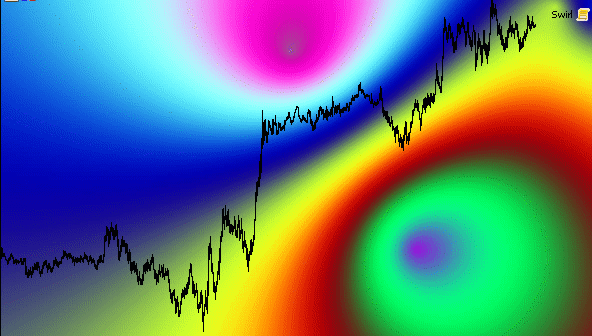```#include <Canvas\Canvas.mqh>

void OnStart()
{
ChartSetInteger(0,CHART_FOREGROUND,true);
CCanvas C;
int Width=(ushort)ChartGetInteger(0,CHART_WIDTH_IN_PIXELS);                               // get Window width
int Height=(ushort)ChartGetInteger(0,CHART_HEIGHT_IN_PIXELS);                             // get Window height
if(!C.CreateBitmapLabel(0,0,"CanvasExamlple",0,0,Width,Height,COLOR_FORMAT_XRGB_NOALPHA)) // create canvas with the size of the current window
Print("Error creating canvas: ",GetLastError());
uint i=0,j=100000;
int size=Width*Height;
uchar h;
for (int w=0;w<25600;w++)
h[w]= uchar(128+128*sin(double(w)/256)); //create an array to speed up the work
double X1=0,Y1=0,X2=0,Y2=0,X3=0,Y3=0,X4=0,Y4=0;
while(!IsStopped())
{
int pos=int(i%size);
if(pos==0)
{
//Sleep(20); //For those who have a too powerful computer.
C.Update();
X1= Width/2-(sin((double)j/100)*(double)Width/2);
Y1= Height/2-(cos((double)j/140)*(double)Height/2);
X2= Width/2+(cos((double)j/80)*(double)Width/2);
Y2= Height/2+(sin((double)j/20)*(double)Height/2);
X3= Width/2+(cos((double)j/85)*(double)Width/2);
Y3= Height/2+(sin((double)j/65)*(double)Height/2);
X4= Width/2+(cos((double)j/152)*(double)Width/2);
Y4= Height/2+(sin((double)j/42)*(double)Height/2);
j++;
}
int X=pos%Width;
int Y=int(pos/Width);
double D1=sqrt((X1-X)*(X1-X)+(Y1-Y)*(Y1-Y));
double D2=sqrt((X2-X)*(X2-X)+(Y2-Y)*(Y2-Y));
double D3=sqrt((X3-X)*(X3-X)+(Y3-Y)*(Y3-Y));
double D4=sqrt((X4-X)*(X4-X)+(Y4-Y)*(Y4-Y));
double d= (D1+D2)/(D1+D2+D3+D4);
//C.m_pixels[pos]=XRGB(h[int(d*11520)],h[int(d*17920)],h[int(d*6400)]);// works a little faster, but requires transferring the array m_pixels from protected to public in Canvas.mqh
C.PixelSet(X,Y,XRGB(h[int(d*11520)],h[int(d*17920)],h[int(d*6400)]));
i++;
}
C.Destroy();
}
```
Files:
LSD.mq5 5 kb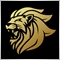Moderator
13108

canvas is capable of everything6758

Marco vd Heijden:
canvas is capable of everything

Absolutely! Even 3D games.40446

You can even use it for useful things.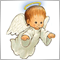3559

Good job :D

But you should rename the script to LSD.mq5 instead of Swirl.mq5 :D11796

Petr Nosek:

Good job :D

But you should rename the script to LSD.mq5 instead of Swirl.mq5 :D

lol6758

Petr Nosek:

Good job :D

But you should rename the script to LSD.mq5 instead of Swirl.mq5 :D

Oh, great idea! Thank you! I'll do it when I get to the computer.6758

With 25 frames
Files:
LSD_25.mq5 6 kb6758

https://www.mql5.com/en/code/22164

I'm sorry if in this moment only in Russian. Google translate will help.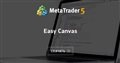Easy Canvas
• www.mql5.com
Данная библиотека и класс iCanvas упростит написание программ с применением Canvas. Вот пример простого индикатора с применением данной библиотеки и его демонстрация. Обратите внимание, что в данном примере в теле индикатора отсутствует функция обработки событий OnChartEvent. Но она также может и присутствовать. Особенности данной библиотеки...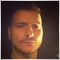21771

Nikolai Semko:

https://www.mql5.com/en/code/22164

I'm sorry if in this moment only in Russian. Google translate will help.

Exceptional work .
I assume you were looking for a faster gradient display which lead to this :).

Files:Moderator
13108

Hey thats prety good :D

Will MetaQuotes take over the Gaming Community ?